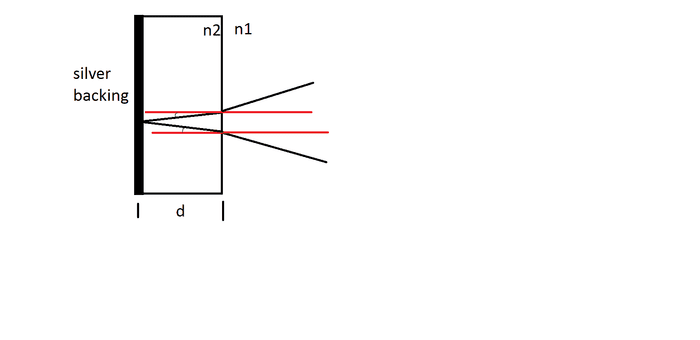# A beam of light makes an angle (how far is the point it leaves the glass from enterin

## Homework Statement

A beam of light makes an angle of 26 o with the normal of a mirror made of 2.4- mm-thick glass silvered on the back. If the index of refraction of the glass is 1.50, how far is the point at which the beam leaves the glass surface (after being reflected from the silver backing) from the point at which the beam entered the glass? ( mm)

## Homework Equations

I thought this was a displacement problem, so these are the equations I used:
Snell's Law: n1sintheta1=n2sintheta2
Displacement: sin(theta2-theta1=d/h

## The Attempt at a Solution

I used h=2.4/sintheta1 to find the hypotenuse after using Snell's law to find theta1 (16.99deg). Then I plugged the numbers into the displacement formula to find d, which I found to be 0.393mm. My homework says this is incorrect, though.

I am completely lost as to how I am going wrong here. Would this not be displacement? This is preparation for my final exam, so if someone could point me in the right direction rather than go over any formulas, that would be appreciated. I want to find the answer myself so I can be sure I know it for the final. Thanks!